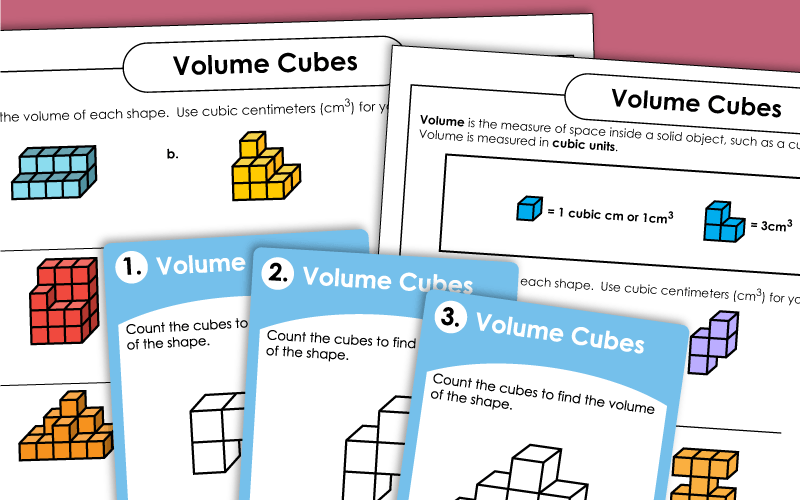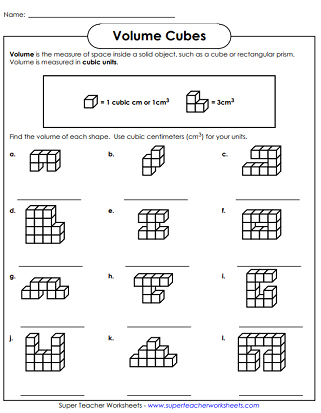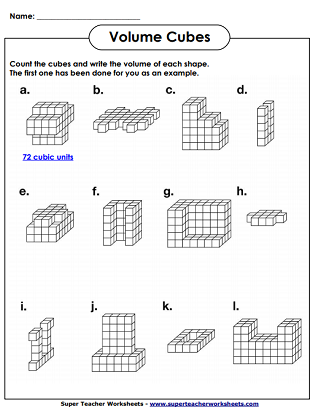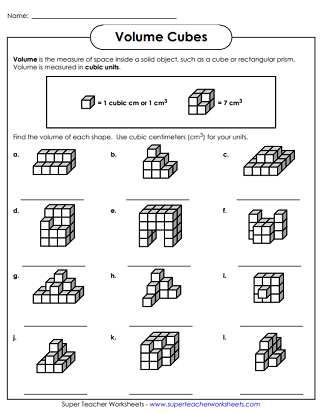# Volume Cubes

Basic worksheets for introducing the concept of volume as cubic units. Students will count the cubes to find the volume of the shapes.

For worksheets on calculating the volumes of solids using measurements and formulas, visit our Volume Worksheets.## Volume CubesBasic

Count the number of cubic units in each shape and write the volume.  There are no hidden cubes on this worksheet.
Print these task cards on card stock and use them for learning centers, classroom scavenger hunts, morning math practice, document camera visuals, or small group instruction.
Count the cubes to find the volume of each shape.  This level does not contain hidden cubes.

## Volume CubesIntermediate

How many cubic units make up each of these shapes?  Count the cubes, but don't forget to include the assumed "hidden" cubes that you can't see.
This file has 25 printable task cards. Figure out the number of cubes that make up each shape to determine the volume in cubic units.
Count the cubes to determine the volume. This level has some cubes that cannot be seen ("hidden cubes").

This worksheet contains large volume cube shapes.  Determine the number of cubic units for each shape.  These solid shapes are very tricky, so students will need to count carefully.
More Volume Worksheets

This page has links to all levels and types of volume worksheets. Calculate the volumes of prisms, cylinders, cones, and spheres.

## Volume Worksheet ImagesMy Account
Site Information